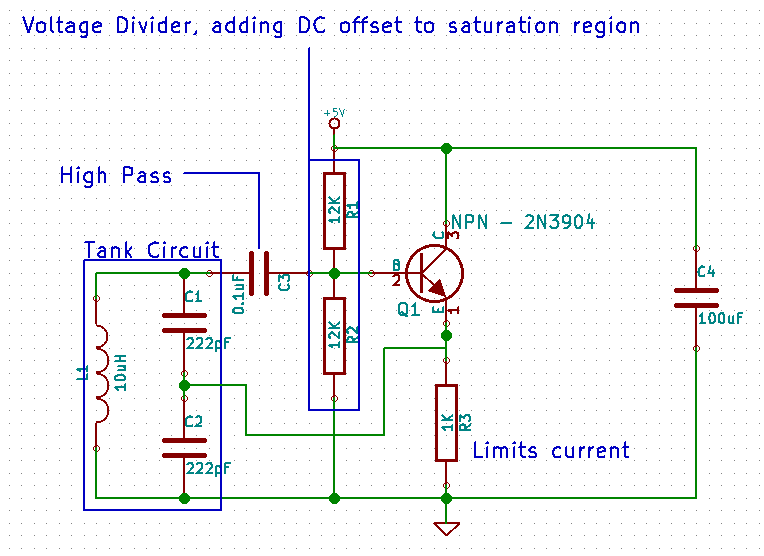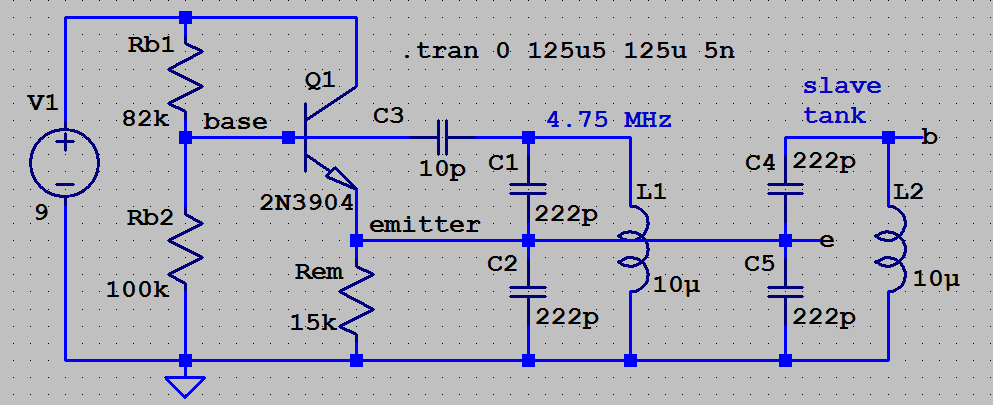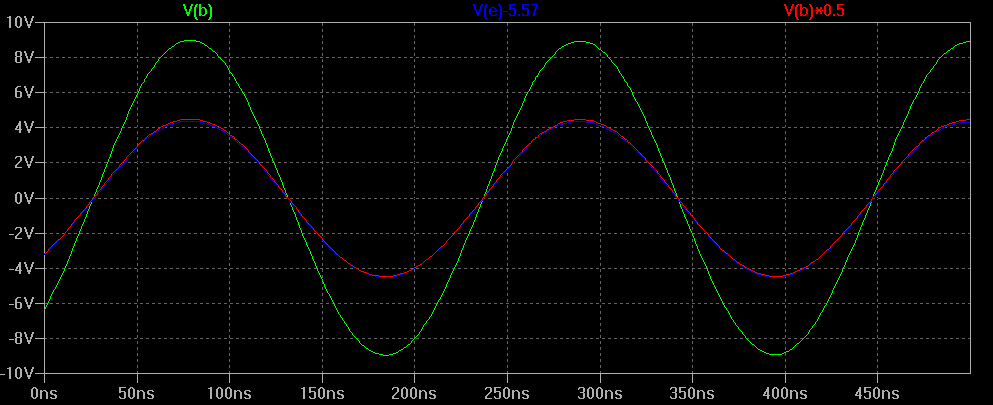# BJT common collector Colpitts oscillator

Summary:
I have trouble finding the amplifier gain of this colpitts oscillator.This is a BJT common collector colpitts oscillator.I have found how to find the feedback fraction from this site:http://fourier.eng.hmc.edu/e84/lectures/ch4/node12.html but I have searched for hours and haven't found the amplifier gain of this circuit.

I have found the amplifier gain for similar colpitts oscillators with a collector resistor and the output of the circuit is the collector voltage but not for this . Help appreciated!

This is a BJT common collector colpitts oscillator.I have found how to find the feedback fraction from this site:http://fourier.eng.hmc.edu/e84/lectures/ch4/node12.html but I have searched for hours and haven't found the amplifier gain of this circuit.

I have found the amplifier gain for similar colpitts oscillators with a collector resistor and the output of the circuit is the collector voltage but not for this . Help appreciated!

Well - the output node of the gain stage in common-collector configuration is - of course - the emitter. But for answering your question one must know which "gain" are you interested in? I suppose, you know that the "open-loop gain" (without the feedback network) is simply Aol=gm*R3/(1+gm*R3), which is very close to unity.
But this is just the most simple part of the problem.
Most important is the loop gain (gain around the closed-loop), which gives you the condition for oscillation and the oscillation frequency.

•DaveE
Well - the output node of the gain stage in common-collector configuration is - of course - the emitter. But for answering your question one must know which "gain" are you interested in? I suppose, you know that the "open-loop gain" (without the feedback network) is simply Aol=gm*R3/(1+gm*R3), which is very close to unity.
But this is just the most simple part of the problem.
Most important is the loop gain (gain around the closed-loop), which gives you the condition for oscillation and the oscillation frequency.
@LvW I know the Barkhausen criteria .I am just interested in the amplifier gain.

Is the load different in the closed loop configuration than the load in the open loop configuration and if so how is it different?

Is the load different in the closed loop configuration than the load in the open loop configuration and if so how is it different?

Is the load different in the closed loop configuration than the load in the open loop configuration and if so how is it different?
Under open-loop conditions the load is given with the emitter resistor only.

DaveE
Gold Member
Under open-loop conditions the load is given with the emitter resistor only.
With the assumption that you've opened the loop between the resistor and the tank, of course. Then when you calculate the closed loop gain you have to account for the extra loading of the amplifier (as defined). This is what you see done in your attachment where they use the Thevenin model with the amp output impedance. In practice, at the resonant frequency, the tank impedance is very high (roughly Q⋅Zc) so it is usually ignored.

BTW, thanks for posting with a nice schematic. It makes it so much easier to understand and communicate about this stuff.

Last edited:
tech99
Gold Member
Summary:: I have trouble finding the amplifier gain of this colpitts oscillator.

View attachment 277764

This is a BJT common collector colpitts oscillator.I have found how to find the feedback fraction from this site:http://fourier.eng.hmc.edu/e84/lectures/ch4/node12.html but I have searched for hours and haven't found the amplifier gain of this circuit.

I have found the amplifier gain for similar colpitts oscillators with a collector resistor and the output of the circuit is the collector voltage but not for this . Help appreciated!
The transistor cannot be said to be in Common Collector.
Look at the AC circuit ignoring DC. The collector load is half the tuned circuit using C2, and it is in parallel with R3. The base-emitter has C1 and the other half of L1.

•DaveE and Helena Wells
Baluncore
The capacitor you show as a high-pass filter is there to block the DC. You can reduce the value of that capacitor to get improved stability. That is because it reduces the amplifier load on the tuned circuit.

I know the Barkhausen criteria .I am just interested in the amplifier gain.
The input impedance differs from the output impedance, so do you want the voltage gain, the current gain or the power gain?

If you post your LTspice.asc.txt file, I can break the loop or parallel your circuit to show model the amplifier gain.

The capacitor you show as a high-pass filter is there to block the DC. You can reduce the value of that capacitor to get improved stability. That is because it reduces the amplifier load on the tuned circuit.

The input impedance differs from the output impedance, so do you want the voltage gain, the current gain or the power gain?

If you post your LTspice.asc.txt file, I can break the loop or parallel your circuit to show model the amplifier gain.
The voltage gain obviously.

DaveE
Gold Member
The voltage gain obviously.
Assuming high transistor gain, and ignoring second order stuff, the voltage gain of an emitter follower is 1. As in post #2.

Assuming high transistor gain, and ignoring second order stuff, the voltage gain of an emitter follower is 1. As in post #2.
ok but what is the impedanve of the load?It isnt just the emitter resistor is it?How C1 and C2 contribute to the impedance of the load?

DaveE
Gold Member
ok but what is the impedanve of the load?It isnt just the emitter resistor is it?How C1 and C2 contribute to the impedance of the load?
At the resonant frequency the tank impedance is very high (since there are essentially no losses shown). So, at that frequency, we would normally assume there is no additional load across the emitter resistor. At other frequencies, or to get an exact solution, is an algebraic nightmare, you have to include lots of parts. You can't just look at C1, or C1 & C2, because all of those parts have similar magnitudes at the frequencies of interest.

For the resonant tank part, if you are far away from resonance, then you will find that either the inductor, or the capacitor can often be ignored because their magnitudes are very different.

At the resonant frequency the tank impedance is very high (since there are essentially no losses shown). So, at that frequency, we would normally assume there is no additional load across the emitter resistor. At other frequencies, or to get an exact solution, is an algebraic nightmare, you have to include lots of parts. You can't just look at C1, or C1 & C2, because all of those parts have similar magnitudes at the frequencies of interest.

For the resonant tank part, if you are far away from resonance, then you will find that either the inductor, or the capacitor can often be ignored because their magnitudes are very different.
What about the parasitic resistance of the inductor/capacitors?

tech99
Gold Member
The transistor cannot be said to be in Common Collector.
Look at the AC circuit ignoring DC. The collector load is half the tuned circuit using C2, and it is in parallel with R3. The base-emitter has C1 and the other half of L1.
The transistor is in Common Emitter. The base-emitter circuit is formed by part of the tuned circuit, and similarly with the collector-emitter circuit. The transistor gain is approx hfe x R3. The Unfortunately, this circuit is very bad because it shunts R3 across the tuned circuit. The Hartley version avoids this issue. There may also be gain due to a step up action by the transformer action of the inductor. It is undesirable to reduce the collector capacitor because this places resistance originating in the collector decoupling resistor across the tuned circuit which lowers the Q. It also creates NFB via R3. If wanting to reduce coupling between tuned circuit and transistor this can be done by tapping the base down the coil (capacitively if wished) or reducing C3.

•Helena Wells
DaveE
Gold Member
Last edited:
•Averagesupernova
DaveE
Gold Member
It is undesirable to reduce the collector capacitor
What collector cap? The 100uF bypassing Vcc to Ground? It sounds like you are looking at a different schematic than me.

•Averagesupernova
DaveE
Gold Member
What about the parasitic resistance of the inductor/capacitors?
Yes, those are real in the practical world, also losses due to radiation. But at 5MHz they shouldn't be very significant, if the components are selected appropriately.

Also the DC bias resistors R1 & R2 are 6Kohm of parallel load on the tank. Since the tank characteristic impedance Zc = √(L/C) = 300 ohm, the 6K in parallel will limit the Q = R/Zc to less than 6K/300 = 20. Also the output impedance of the amplifier (R3), etc. The exact solution is complex.

These circuits are almost always analyzed by making some assumptions to simplify things. Like ignoring the loading of R1 & R2 because Q=20 is almost the same as Q=∞. We know those assumptions are approximations, once you get a simplified model looking OK, then you can go back and see if you really do care about some of those details we skipped over.

Last edited:
Baluncore
The voltage gain obviously.
The BJT is an emitter follower with a voltage gain slightly less than one.

Model another tank circuit, identical to L1, C2 and C3. Connect the lower end of L2 to ground. Connect the centre of the two capacitors to the same emitter drive terminal on the existing modelled oscillator. The simulation should run with the tank circuit slaved in parallel without any big change to the simulation.

The top of the slave, L2 is disconnected from the base terminal. Look at the sine wave on that terminal and compare it to the emitter voltage. You will see that for C1=C2, the voltage ratio is exactly two.

The L1-C1-C2 tank acts like a centre tapped transformer winding = an autotransformer. That provides the voltage gain of two required to sustain oscillation.

The BJT is a buffer amplifier that converts a high impedance at the base terminal, to the low impedance at the emitter terminal. In effect it has a current gain.

Baluncore
The emitter resistor can be increased to 15 kΩ, and there is less distortion with a higher base bias impedance and a smaller feedback capacitor = 10 pF.

The base impedance is then about 40 kΩ. The output impedance of the emitter follower is about Re / β ≈ 100 Ω.

Here is the schematic with the plot of base and emitter voltages.Note that Ve with offset is plotted in blue, over Vb/2 in red.tech99
Gold Member
I have attached a diagram showing how the circuit may be regarded as common emitter.

#### Attachments

• colpitts.pdf
121 KB · Views: 33
•alan123hk
Baluncore
I have attached a diagram showing how the circuit may be regarded as common emitter.
I am not convinced. The transistor is operating as an emitter-follower, which really makes it a common collector configuration. The base is the input, the emitter is the output.

tech99
Gold Member
I am not convinced. The transistor is operating as an emitter-follower, which really makes it a common collector configuration. The base is the input, the emitter is the output.
I think people are assuming emitter follower because the circuit is drawn with the collector grounded for RF. That does not make it common collector. The ground can be placed anywhere on an oscillator circuit.

I think people are assuming emitter follower because the circuit is drawn with the collector grounded for RF. That does not make it common collector. The ground can be placed anywhere on an oscillator circuit.
No - we are not "assuming" something. The circuit is indeed an emitter follower - the collector is at signal ground and the emitter potential is "following" (with a very small error) the varying base potential. What else do we need to call it correctly "emitter follower"?
And yes - you are right. We can make use of the "virtual ground" concept as proposed by Alechno long time ago. This is a good method to convert the shown circuit into a common emitter stage (gain>1) with a frequency-dependent positive feedback path. In some cases, such a conversion can help to better understand the function of some circuits.
But without such ground shifting the given circuit remains what it is: A common collector stage (emitter follower).

DaveE